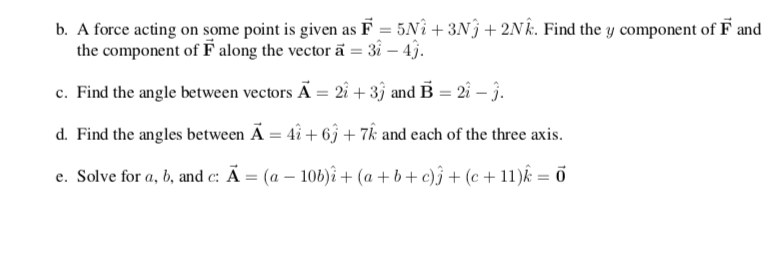Home / Answered Questions / Other / b-a-force-acting-on-some-point-is-given-as-f-5ni-3n9-2n-k-find-the-y-component-of-f-and-the-componen-aw187

# (Solved): B. A Force Acting On Some Point Is Given As F = 5NÃ® + 3N9 + 2N K. Find The Y Component Of F And The...ALL PARTS PLEASE

b. A force acting on some point is given as F = 5NÃ® + 3N9 + 2N k. Find the y component of F and the component of F along the vector Ä= 3i - 4j. c. Find the angle between vectors A = 2Ã®+3j and B = 2Ã® - . d. Find the angles between A = 4 + 6 + 7k and each of the three axis. e. Solve for a, b, and c: A = (a - 106)áº£ + (a +b+c) Ã®+(c +11)k = 7

We have an Answer from Expert